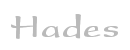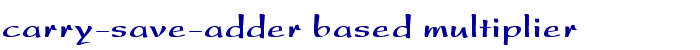TAMS / Java / Hades / applets (print version): contents | previous | next

#### Carry-save-adder based serial multiplier (6x6 bits)Description

This circuit demonstrates a 6x6 bits serial multiplication using a carry-save adder. First, enter the input values for the A and B operand registers. Just click into the corresponding bit position in the registers to toggle the value. Just play with several values for the input operands. An interesting set of input values is A=111111 and B=111111, to give the maximum result value.

Second, switch the start input of the controller state machine to 1, which tells the controller to start a new multiplication.

Next, toggle the clock input to step through the multiplication cycles. During each cycle, one bit of operand A is read and multiplied with operand B (via 6 AND gates) to give a partial product. This partial product is then added via the carry-save adder with the previous partial product and carries, resulting in a new partial product and carries.

Also, the operand A register is shifted 1-bit right, so that the next step uses the next bit of A. In this demonstration, the freed bit positions of register A are filled with X values, to indicate which values of A have already been used. (The software implementation on a processor could use a rotate A instruction instead of shift-right, so that the value of A is restored after all bits of A have been used.)

After six steps of the algorithm, the standard adder is used to add the last set of carries and partial product values to give the final multiplication result. Note: Naturally, using a carry-save adder is not really useful in a clocked serial multiplier, because the clock frequency is limited by the slowest component and the carry-save adder does not result in a performance advantage. The usual application of carry-save adders is in a combinatorical (Wallace-tree) implementation of multipliers.

Run the applet | Run the editor (via Webstart)

Impressum | 24.11.06